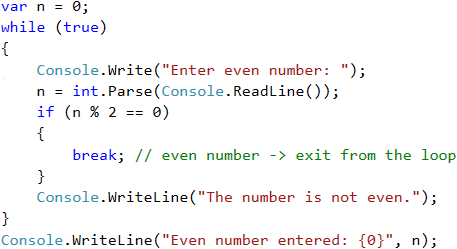# Example: Enter an Even Number

The next example will be to write a program that enters an even number from the console. If an odd number is entered, the program should enter a number again, until an even number is entered.

We shall use an infinite loop with break to solve this problem, because we don't know how many times the loop body will be repeated.

We shall check if a particular number n is even, and if it is, we will print it on the screen. An even number is one that can be divided by 2 without remainder. If an invalid number is entered, we will ask the user to enter a number again and will display a notification that the input number is not even.

## Hints and Guidelines

Here is an idea how we can implement the above described logic:

• We create a variable n to which we assign an initial value of 0.
• We create an infinite while loop and as condition we will set true.
• In the body of the loop:
• We take an integer value from the console input and assign it to n.
• If the number is even, we exit the loop by break.
• Otherwise, we display a message stating that the number is not even. The iterations continue until an even number is entered.
• Finally, after the loop, print the even number on the screen.

## Implementation

Here is an example implementation of the idea:Note: Although the code above is correct, it will not work if the user enters text instead of numbers, such as "Invalid number". Then parsing the text to a number will break and the program will display an error message (exception). How to deal with this problem and how to capture and process exceptions using the try-catch construction will be learned later.